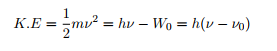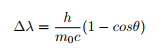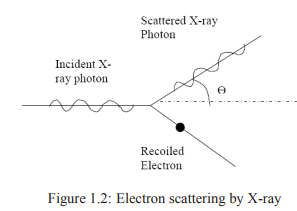Courses

# Photoelectric Effect Civil Engineering (CE) Notes | EduRev

## Civil Engineering (CE) : Photoelectric Effect Civil Engineering (CE) Notes | EduRev

The document Photoelectric Effect Civil Engineering (CE) Notes | EduRev is a part of Civil Engineering (CE) category.
All you need of Civil Engineering (CE) at this link: Civil Engineering (CE)

Photoelectric effect
The phenomenon of emission of electrons by the surface of certain materials under the influence of radiation of suitable frequency is called photoelectric effect.
The materials which exhibit this property are called photosensitive while the electrons emitted are called photoelectrons.

The following observations are made during the experimental study of photoelectric effect.
1. Photoelectric effect is an instantaneous process. i.e., electrons are emitted by the surface as soon as radiation falls on the surface.
2. If frequency of the incident radiation is less than certain value, photoelectrons are not emitted however strong the intensity of the incident radiation may be. The frequency above which photoelectric effect takes place is called threshold frequency denoted by νo.
3. Kinetic energy of the photoelectrons emitted is directly proportional to the frequency of the incident radiation and independent of the intensity of radiation.
4. Number of photoelectrons emitted is directly proportional to the intensity of the incident radiation but independent of the frequency of the radiation.
5. For a particular value of negative potential given to anode, photoelectric current becomes zero. It is called stopping potential denoted by Vs. Kinetic energy of photoelectrons expressed in eV gives stopping potential

Einstein’s explanation of photoelectric effect
Free electrons in metal are bound within the surface of the metal. Minimum energy required for the electron to escape from the surface of the metal is called Work Function and is denoted by W0. According to Einsteins quantum theory of light, beam of light consists of stream of particles called quanta. The energy of each quanta which is also called as photon is given by E = hν , where h is Plancks constant and ν is frequency of the radiation. When light radiation of frequency (> ν0) is made to fall on the surface of the metal, photon of the light interacts with electrons and transfers its energy completely to the electron. Since energy hν absorbed by the electron is greater than the work function W0 = hν0, electron escape from the surface of the metal leading to photoelectric emission. According to the law of conservation of energy, kinetic energy of the electrons emitted is given byThe above equation is called Einsteins photo-electric equation.
All the experimental results of photoelectric effect can be explained using above equation.

Compton Effect
The phenomenon in which the wavelength of X-rays increases when scattered from a suitable target is called Compton Effect.This kind of scattering is also called incoherent scattering.

Explanation:
When x-ray photon interacts with matter, it collides with electrons and transfers its energy partially to the electron and get scattered with lesser energy. As a result frequency of the scattered x-ray is less than the incident beam and hence wavelength of the scattered beam is more than that of the incident beam. It can be shown that change in wavelength when X-ray undergo Compton scattering is given byWhere h is Plancks constant, m0 is rest mass of the electron; c is velocity of light in free space and θ is the angle of scattering.
∆λ is called Compton Shift (h/m0c) and is called Compton wavelength.Offer running on EduRev: Apply code STAYHOME200 to get INR 200 off on our premium plan EduRev Infinity!

,

,

,

,

,

,

,

,

,

,

,

,

,

,

,

,

,

,

,

,

,

;